打赏

# 《算法图解》第七章笔记_迪杰斯特拉算法

## 一、迪杰斯特拉（dijkstras）算法介绍

1. 找出最短时间内前往的节点；
2. 对于该节点的邻居，检查是否有前往它们的更短路径，如果有，就更新其开销；
3. 重复这个过程，直到对图中的每个节点都重复了以上两个步骤；
4. 计算最终路径。

## 二、迪杰斯特拉算法术语介绍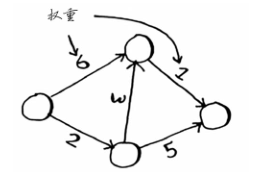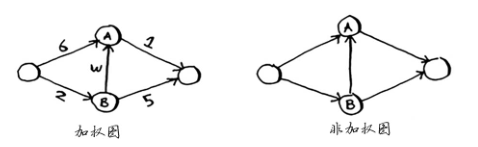## 三、算法实现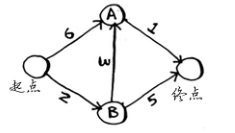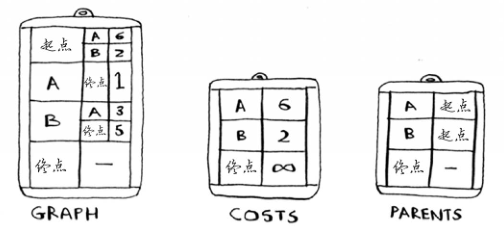graph = {}  #首先需要实现这个图

graph["start"] = {}
graph["start"]["a"] = 6
graph["start"]["b"] = 2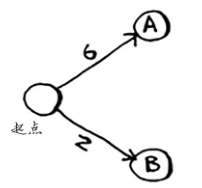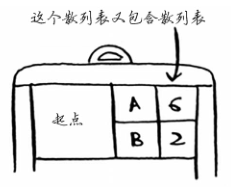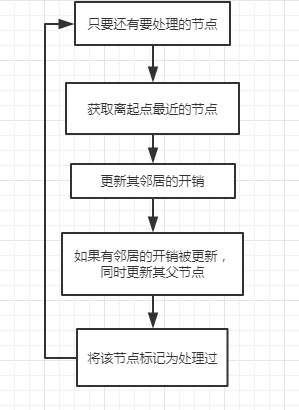# 添加节点和邻居
graph = {}
graph["start"] = {}
graph["start"]["a"] = 6
graph["start"]["b"] = 2

graph["a"] = {}
graph["a"]["fin"] = 1

graph["b"] = {}
graph["b"]["a"] = 3
graph["b"]["fin"] = 5

graph["fin"] = {}  # 终点没有邻居

# 存储每个节点开销的散列表
infinity = float("inf")
costs = {}
costs["a"] = 6
costs["b"] = 2
costs["fin"] = infinity

# 存储父节点的散列表
parents = {}
parents["a"] = "start"
parents["b"] = "start"
parents["fin"] = None

processed = []  # 一个数组，用于记录处理过的节点。因为对于同一个节点，不用处理多次。

def find_lowest_cost_node(costs):
lowest_cost = float("inf")
lowest_cost_node = None
# 遍历所有的节点
for node in costs:
cost = costs[node]
# 如果当前节点的开销更低且未处理过
if cost < lowest_cost and node not in processed:
# 就将其视为开销最低的节点
lowest_cost = cost
lowest_cost_node = node
return lowest_cost_node

# 在未处理的节点中找出开销最小的节点
node = find_lowest_cost_node(costs)
# 这个while循环在所有节点都被处理过后结束
while node is not None:
cost = costs[node]
# 遍历当前节点的所有邻居
neighbors = graph[node]
for n in neighbors.keys():
new_cost = cost + neighbors[n]
# 如果经当前节点前往该邻居更近
if costs[n] > new_cost:
# 就更新该邻居的开销
costs[n] = new_cost
# 同时将该邻居的父节点设置为当前节点
parents[n] = node
# 将当前节点标记为处理过
processed.append(node)
# 找出接下来要处理的节点，并做循环
node = find_lowest_cost_node(costs)

print ("Cost from the start to each node:")
print (costs)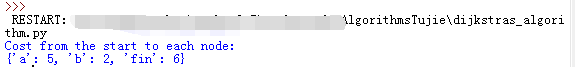## 四、小结

• 广度有限搜索用于在非加权图中查找最短路径。
• 迪杰斯特拉算法用于在加权图中查找最短路径。
• 仅当权重为正时迪杰斯特拉算法才管用。
• 如果图中包含负权边，考虑使用贝尔曼-福德（Bellman-Ford）算法。

posted @ 2018-06-11 18:02  Zoctopus_Zhang  阅读(3845)  评论(0编辑  收藏
// function btn_donateClick() { var DivPopup = document.getElementById('Div_popup'); var DivMasklayer = document.getElementById('div_masklayer'); DivMasklayer.style.display = 'block'; DivPopup.style.display = 'block'; var h = Div_popup.clientHeight; with (Div_popup.style) { marginTop = -h / 2 + 'px'; } } function MasklayerClick() { var masklayer = document.getElementById('div_masklayer'); var divImg = document.getElementById("Div_popup"); masklayer.style.display = "none"; divImg.style.display = "none"; } setTimeout( function () { document.getElementById('div_masklayer').onclick = MasklayerClick; document.getElementById('btn_donate').onclick = btn_donateClick; var a_gzw = document.getElementById("guanzhuwo"); a_gzw.href = "javascript:void(0);"; \$("#guanzhuwo").attr("onclick","follow('33513f9f-ba13-e011-ac81-842b2b196315');"); }, 900);